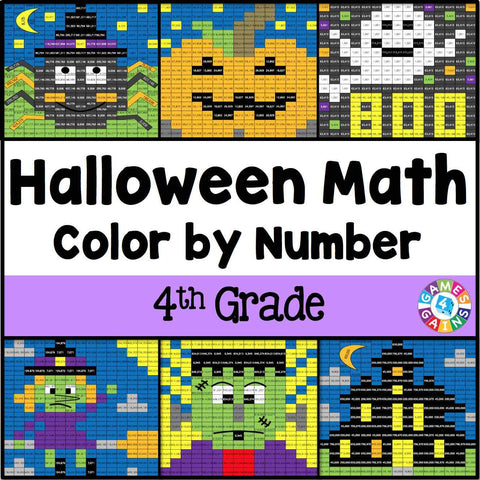## Halloween Math Color-by-Number - 4th Grade

• \$480

Halloween Math Color-by-Number set comes with 6 Halloween math color-by-number activities for reviewing 4th grade math skills. This Halloween math set is perfect to use in centers, in small groups, or with the whole class!

Included with this Halloween Math Color-by-Number set:
Instructions
6 color by number worksheets
6 student problem worksheets
6 teacher answer sheets

Here are the skills covered by these Halloween math color-by-number activities:

• Writing numbers within 1,000,000 in standard form from expanded form and word form
• Comparing numbers within 1,000,000
• Rounding numbers within 1,000,000
• Adding and subtracting numbers within 1,000,000 using the standard algorithm
• Adding and subtracting numbers within 1,000,000 in one-step word problems
• Adding and subtracting numbers within 1,000,000 in two-step word problems

Want to learn more about what you'll get? Check out the preview!